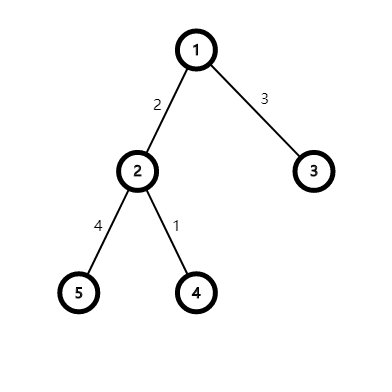# Tree

You are given a weighted undirected tree T of N nodes. Each edge i has a weight .

Assume that the edges you need to travel from node i to j is
then we define

For each node i, print

# Example

Tree={
1->2,2
1->3,3
2->4,1
2->5,4
}

-4 2 13 -1 2
Explain:For node 1,0+2+3-6-3=-4
For node 2,2+0-5+1+4=2
For node 3,3-5+0+6+9=13
For node 4,-3+1+6+0-5=-1
For node 5,-6+4+9-5+0=2